# Feedback Control of a ROS-Enabled Robot Over ROS 2

This example shows you how to use Simulink® to control a simulated robot running in a Gazebo® robot simulator over ROS 2 network.

### Introduction

In this example, you will run a model that implements a simple closed-loop proportional controller. The controller receives location information from a simulated robot and sends velocity commands to drive the robot to a specified location. You will adjust some parameters while the model is running and observe the effect on the simulated robot.

The following diagram summarizes the interaction between Simulink and the robot simulator (the arrows in the diagram indicate ROS 2 message transmission). The `/odom` topic conveys location information, and the `/cmd_vel` topic conveys velocity commands.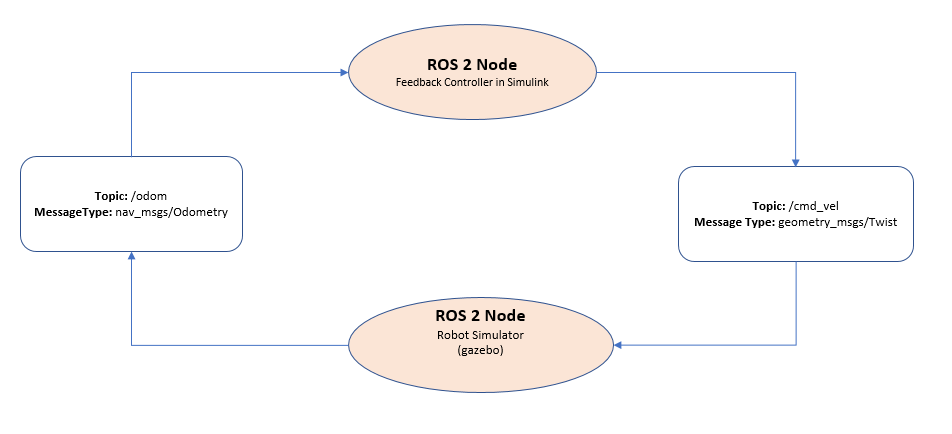### Start a Robot Simulator and Configure Simulink

In this task, you will start a ROS-based simulator for a differential-drive robot, start the ROS bridge configure MATLAB® connection with the robot simulator.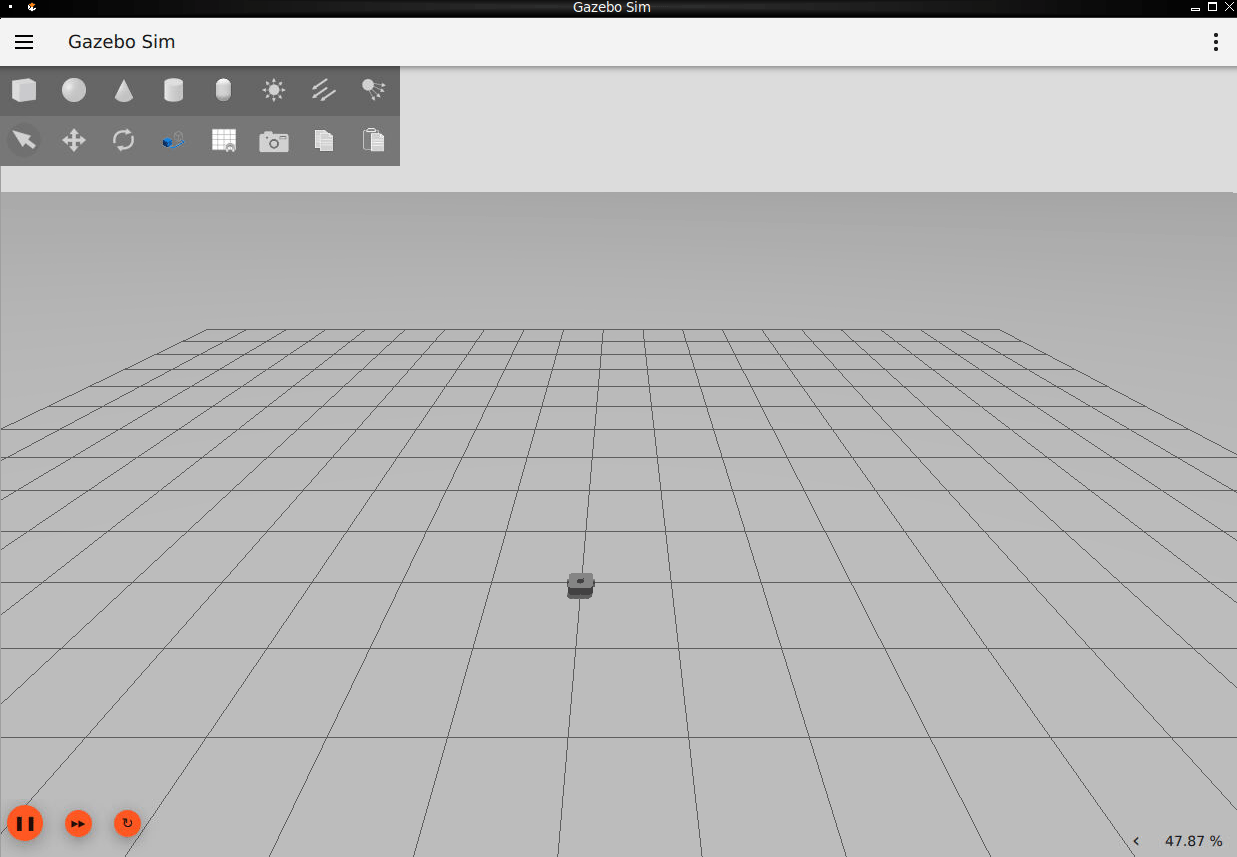• In the Ubuntu desktop, click the Gazebo Empty icon to start the empty Gazebo world.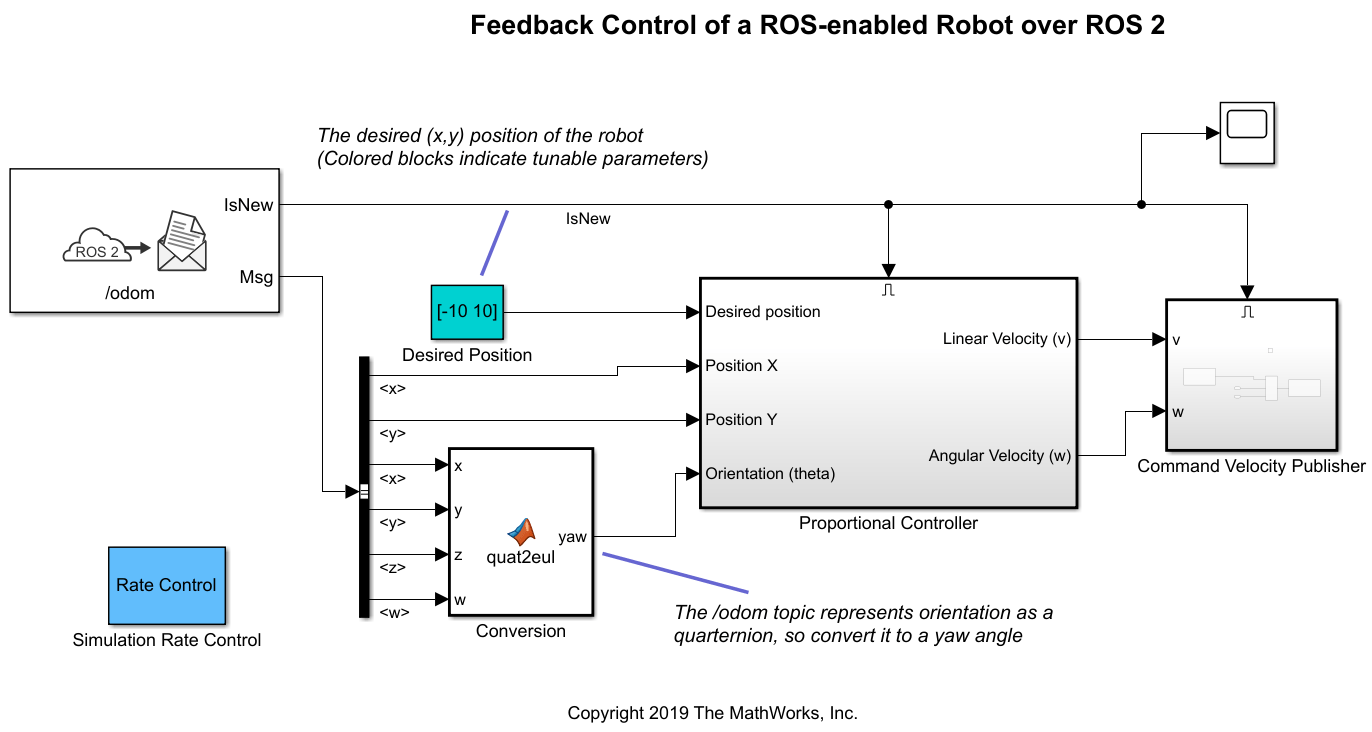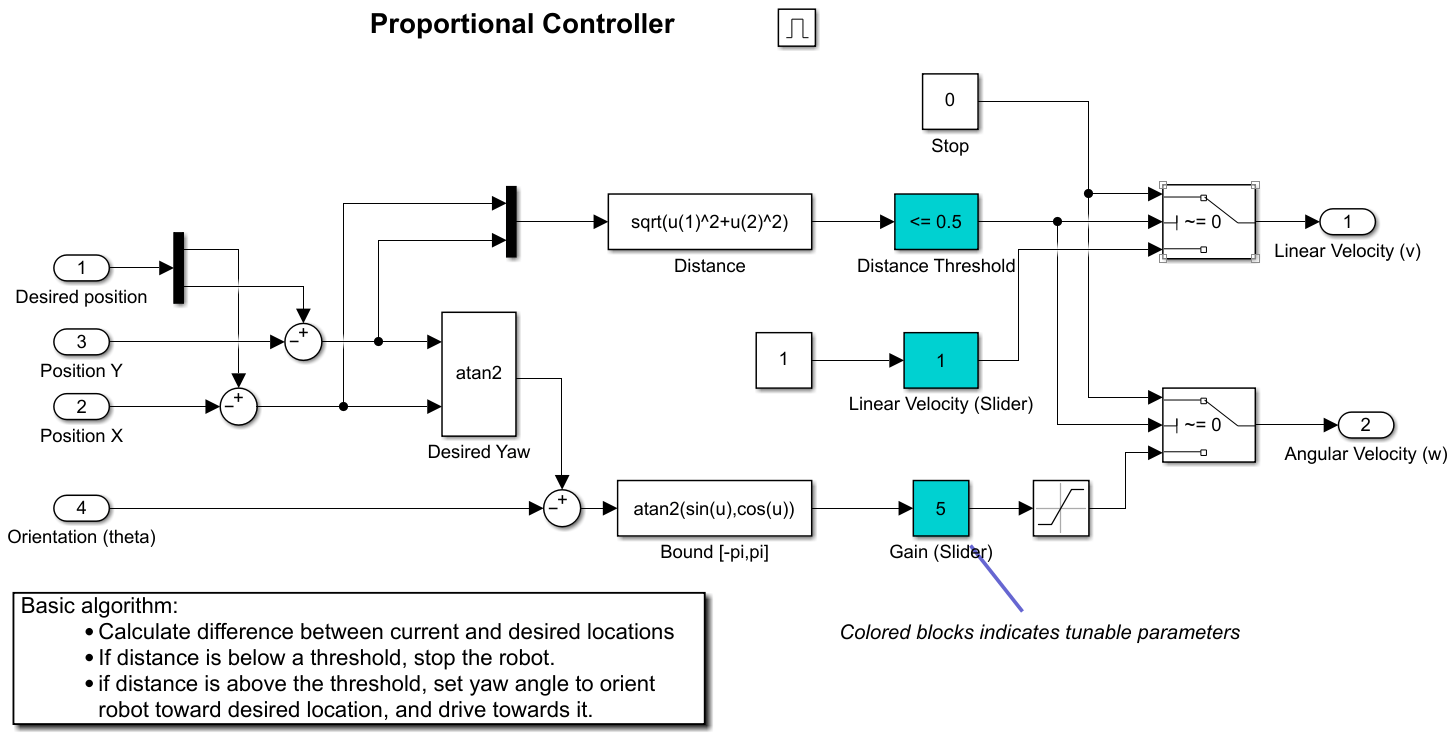• Click the ROS Bridge icon to start the ROS bridge to relay messages between Simulink ROS 2 node and Turtlebot3 ROS-enabled robot.

• In MATLAB on your host machine, set the proper domain ID for the ROS 2 network using the `'ROS_DOMAIN_ID'` environment variable to `25` to match the robot simulator ROS bridge settings and run `ros2 topic list` to verify that the topics from the robot simulator are visible in MATLAB.

```setenv('ROS_DOMAIN_ID','25') ros2 topic list```
```/clock /gazebo/link_states /gazebo/model_states /gazebo/performance_metrics /imu /joint_states /odom /parameter_events /rosout /rosout_agg /scan /tf ```

### Open Existing Model

After connecting to the ROS 2 network, open the example model.

`open_system('robotROS2FeedbackControlExample.slx');`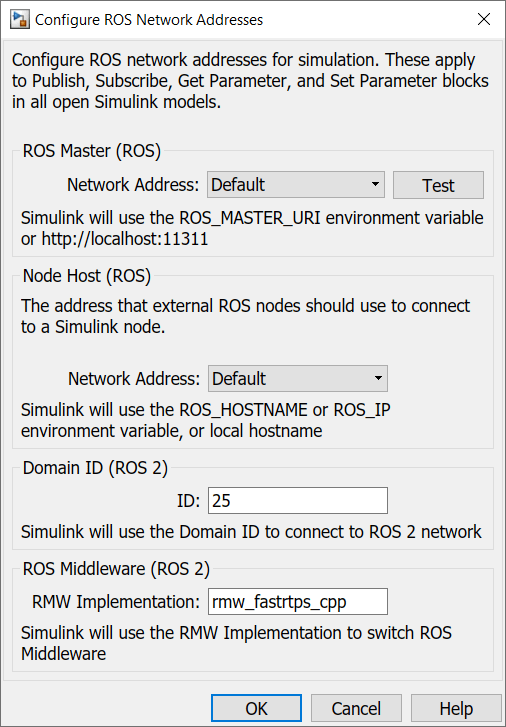The model implements a proportional controller for a differential-drive mobile robot. On each time step, the algorithm orients the robot toward the desired location and drives it forward. Once the desired location is reached, the algorithm stops the robot.

`open_system('robotROS2FeedbackControlExample/Proportional Controller');`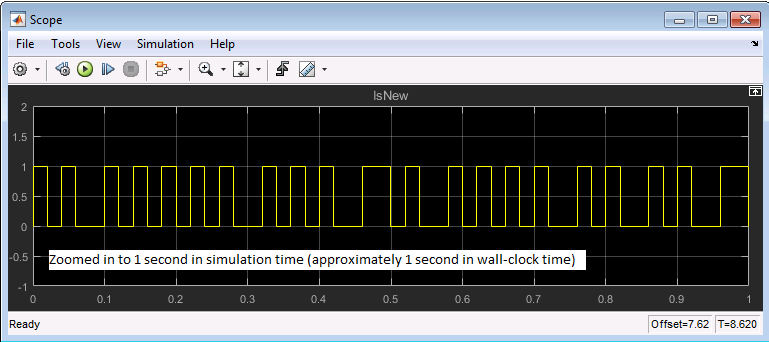Note that there are four tunable parameters in the model (indicated by colored blocks).

• Desired Position (at top level of model): The desired location in (X,Y) coordinates

• Distance Threshold: The robot is stopped if it is closer than this distance from the desired location

• Linear Velocity: The forward linear velocity of the robot

• Gain: The proportional gain when correcting the robot orientation

The model also has a Simulation Rate Control block (at top level of model). This block ensures that the simulation update intervals follow wall-clock elapsed time.

### Configure Simulink and Run the Model

In this task, you will configure Simulink to communicate with ROS-enabled robot simulator over ROS 2, run the model and observe the behavior of the robot in the robot simulator.

To configure the network settings for ROS 2.

• Under the Simulation tab, in PREPARE, select ROS Toolbox > ROS Network.

• In Configure ROS Network Addresses, set the ROS 2 Domain ID value to 25 and set the ROS 2 RMW Implementation as `rmw_fastrtps_cpp`.

• Click OK to apply changes and close the dialog.To run the model.

• Position windows on your screen so that you can observe both the Simulink model and the robot simulator.

• Click the Play button in Simulink to start simulation.

• While the simulation is running, double-click on the Desired Position block and change the Constant value to `[2 3]`. Observe that the robot changes its heading.

• While the simulation is running, open the Proportional Controller subsystem and double-click on the Linear Velocity (slider) block. Move the slider to 2. Observe the increase in robot velocity.

• Click the Stop button in Simulink to stop the simulation.

### Observe Rate of Incoming Messages

In this task, you will observe the timing and rate of incoming messages.

• Click the Play button in Simulink to start simulation.

• Open the Scope block. Observe that the IsNew output of the Subscribe block is always zero, indicating that no messages are being received for the /odom topic. The horizontal axis of the plot indicates simulation time.

• Start Gazebo Simulator in ROS network and start ROS Bridge in ROS 2, so that ROS 2 network able receive messages published by Gazebo Simulator.

• In the Scope display, observe that the IsNew output has the value 1 at an approximate rate of 20 times per second, in elapsed wall-clock time.The synchronization with wall-clock time is due to the Simulation Rate Control block. Typically, a Simulink simulation executes in a free-running loop whose speed depends on complexity of the model and computer speed (see Simulation Loop Phase (Simulink)). The Simulation Rate Control block attempts to regulate Simulink execution so that each update takes 0.02 seconds in wall-clock time when possible (this is equal to the fundamental sample time of the model). See the comments inside the block for more information.

In addition, the Enabled subsystems for the Proportional Controller and the Command Velocity Publisher ensure that the model only reacts to genuinely new messages. If enabled subsystems were not used, the model would repeatedly process the same (most-recently received) message over and over, leading to wasteful processing and redundant publishing of command messages.

### Summary

This example showed you how to use Simulink for simple closed-loop control of a simulated robot. It also showed how to use Enabled subsystems to reduce overhead in the ROS 2 network.